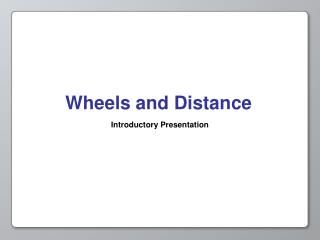DownloadDownload PresentationWheels and Distance

# Wheels and Distance

Download Presentation## Wheels and Distance

- - - - - - - - - - - - - - - - - - - - - - - - - - - E N D - - - - - - - - - - - - - - - - - - - - - - - - - - -
##### Presentation Transcript

1. Wheels and Distance Introductory Presentation

2. Opening Activity In “Full Speed Ahead” we made our robot move forward with the program below.

3. Opening Activity In this program: 1. How far did your robot move forward? 2. What affected the distance it moved? 3. How could you get it to move a longer distance? A shorter distance? An exact distance you want?

4. Opening Activity Many things affected how far your robot traveled. • The number of degrees your Wait For block is set to wait for • The size of your tires

5. Wheels and Distance In this activity we’re going to program our robot to move an exact distance. To do so we must understand a few things about circles. START FINISH

6. r d Review Let’s start with the basics. Answer the following: The distance from the center to the outside of a circle. 1. What is a radius of a circle? 2. What is a diameter of a circle? 3. What is the formula for the circumference of a circle? The distance, through a circle’s center, from one edge to another. Circumference= diameter * π

7. Preview With our knowledge of circumference, we can start figuring out how to control the distance our robot goes.

8. Preview First, we need to understand that the circumference of a circle is really just a line curled around a center point.

9. d = 4 Review From this, we know that the circumference of a circle and the length of the line it rolls out are equal in length. Finish the following: Circumference = 4 * π So we know that… Length of Line = 4 * π

10. Preview Let’s try a few more. Finish this chart: Circumference = Length of line = 1 meter 1 meter 2 meters 2 meters 7 meters 7 meters

11. Preview Predict what would happen if we were to change the number of rotations that the wheel makes? Or change the diameter of the wheel? Once you have completed the activity check your prediction

12. Preview In the meantime, let’s review a few more topics that you will need to successfully complete the Wheels and Distance Investigation.

13. Review: Average To find the average of a collection of measurements: • Add all of your measurements together • Divide by the number of measurements Measurement 1 + Measurement 2 + Measurement 3 Average = 3

14. Review: Average Suppose your robot moved forward three times with distances of 5, 6, and 10 meters. What is the average distance it moved forward? + + Measurement 1 5 Measurement 2 6 Measurement 3 10 Average = 7 meters # of measurements 3

15. Review: Degrees 360˚ 0˚ 270˚ 90˚ 180˚ There are 360˚ in one full rotation of a wheel

16. Review: Degrees If there are 360˚ in one rotation of a wheel, how many degrees are there in two rotations? Answer: 720˚ 720˚ = 2 rotations * 360˚ Go to the next page for more practice!

17. Review: Degrees Complete the following chart to get some practice: # Of Rotations # of Degrees 1 rot. 360˚ 3 rot. 1080˚ 1.5 rot. 540˚

18. Review Keep the following in mind as you complete the Wheels and Distance Investigation: • Circumference - the length of line a circle rolls out • Circumference = diameter * π • Average = sum of measurements number of measurements • There are 360˚ in one rotation of a circle

19. Review: Scientific Inquiry Scientific Inquiry This is the process by which people ask questions, and seek out answers to their questions through the use of experiments and scientific knowledge.

20. Review: Scientific Inquiry Hypothesis A key element to Scientific Inquiry is the scientist's hypothesis. A hypothesis is an educated guess concerning the outcome of the inquiry process and the experiments conducted within.

21. Good Luck! Now you have the necessary knowledge to get started in the Wheels and Distance Investigation.